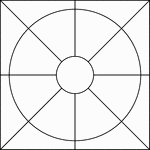### Geometric Block Pattern 69

Geometric pattern for translation and rotation exercises.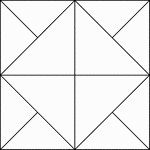### Geometric Block Pattern 7

Geometric pattern for translation and rotation exercises.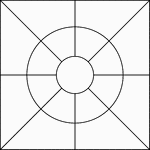### Geometric Block Pattern 70

Geometric pattern for translation and rotation exercises.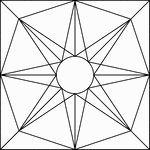### Geometric Block Pattern 71

Geometric pattern for translation and rotation exercises.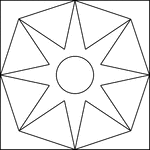### Geometric Block Pattern 72

Geometric pattern for translation and rotation exercises.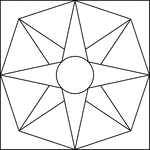### Geometric Block Pattern 73

Geometric pattern for translation and rotation exercises.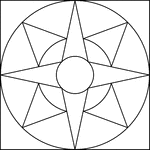### Geometric Block Pattern 74

Geometric pattern for translation and rotation exercises.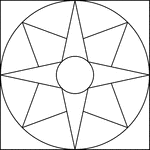### Geometric Block Pattern 75

Geometric pattern for translation and rotation exercises.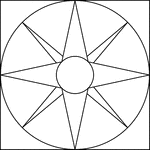### Geometric Block Pattern 76

Geometric pattern for translation and rotation exercises.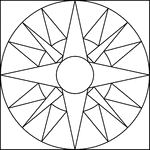### Geometric Block Pattern 77

Geometric pattern for translation and rotation exercises.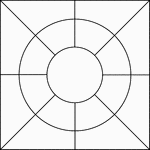### Geometric Block Pattern 78

Geometric pattern for translation and rotation exercises.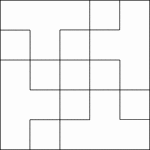### Geometric Block Pattern 79

Geometric pattern for translation and rotation exercises.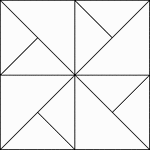### Geometric Block Pattern 8

Geometric pattern for translation and rotation exercises.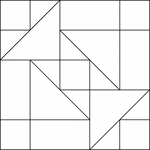### Geometric Block Pattern 80

Geometric pattern for translation and rotation exercises.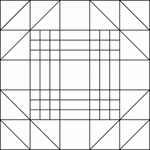### Geometric Block Pattern 81

Geometric pattern for translation and rotation exercises.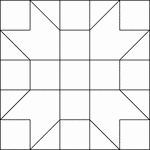### Geometric Block Pattern 82

Geometric pattern for translation and rotation exercises.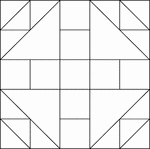### Geometric Block Pattern 83

Geometric pattern for translation and rotation exercises.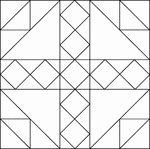### Geometric Block Pattern 84

Geometric pattern for translation and rotation exercises.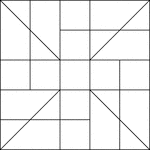### Geometric Block Pattern 85

Geometric pattern for translation and rotation exercises.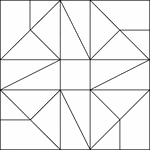### Geometric Block Pattern 86

Geometric pattern for translation and rotation exercises.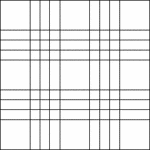### Geometric Block Pattern 87

Geometric pattern for translation and rotation exercises.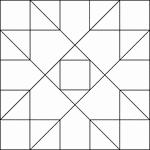### Geometric Block Pattern 88

Geometric pattern for translation and rotation exercises.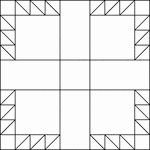### Geometric Block Pattern 89

Geometric pattern for translation and rotation exercises.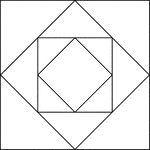### Geometric Block Pattern 9

Geometric pattern for translation and rotation exercises.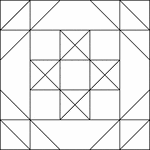### Geometric Block Pattern 90

Geometric pattern for translation and rotation exercises.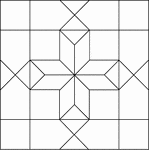### Geometric Block Pattern 91

Geometric pattern for translation and rotation exercises.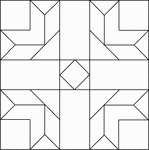### Geometric Block Pattern 92

Geometric pattern for translation and rotation exercises.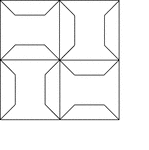### Geometric Block Pattern 93

Geometric pattern for translation and rotation exercises.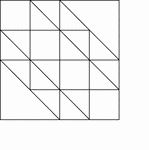### Geometric Block Pattern 94

Geometric pattern for translation and rotation exercises.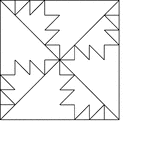### Geometric Block Pattern 95

Geometric pattern for translation and rotation exercises.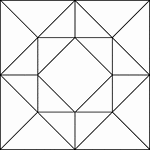### Geometric Block Pattern 96

Geometric pattern for translation and rotation exercises.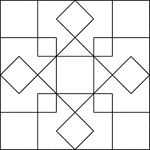### Geometric Block Pattern 97

Geometric pattern for translation and rotation exercises.### Geometric Block Pattern 98

Geometric pattern for translation and rotation exercises.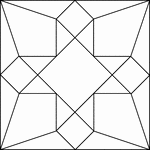### Geometric Block Pattern 99

Geometric pattern for translation and rotation exercises.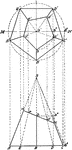### Frustum of Pentagonal Pyramid

Illustration of a frustum of a pentagonal pyramid (including cross-section).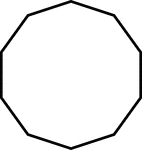### 10-sided Polygon

Polygon consisting of 10 sides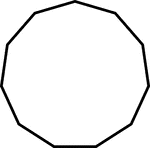### 11-sided Polygon

Polygon consisting of 11 sides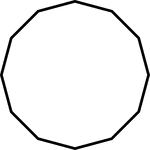### 12-sided Polygon

Polygon consisting of 12 sides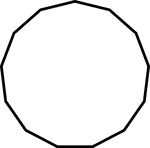### 13-sided Polygon

Polygon consisting of 13 sides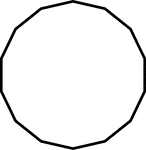### 14-sided Polygon

Polygon consisting of 14 sides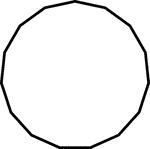### 15-sided Polygon

Polygon consisting of 15 sides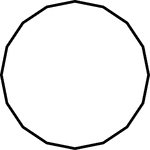### 16-sided Polygon

Polygon consisting of 16 sides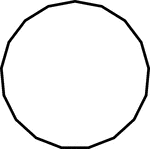### 17-sided Polygon

Polygon consisting of 17 sides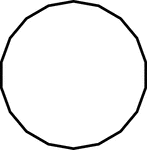### 18-sided Polygon

Polygon consisting of 18 sides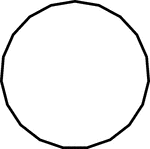### 19-sided Polygon

Polygon consisting of 19 sides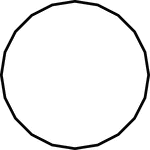### 20-sided Polygon

Polygon consisting of 20 sides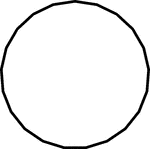### 21-sided Polygon

Polygon consisting of 21 sides### 22-sided Polygon

Polygon consisting of 22 sides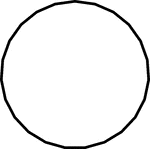### 23-sided Polygon

Polygon consisting of 23 sides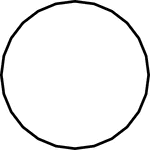### 24-sided Polygon

Polygon consisting of 24 sides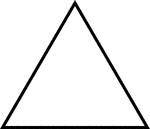### 3-sided Polygon

Polygon consisting of 3 sides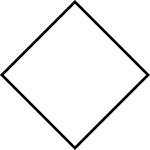### 4-sided Polygon

Polygon consisting of 4 sides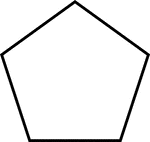### 5-sided Polygon

Polygon consisting of 5 sides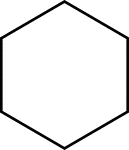### 6-sided Polygon

Polygon consisting of 6 sides### 7-sided Polygon

Polygon consisting of 7 sides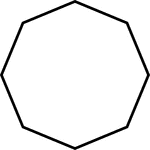### 8-sided Polygon

Polygon consisting of 8 sides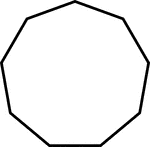### 9-sided Polygon

Polygon consisting of 9 sides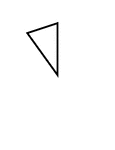### Fractions of 10-sided Polygon

1/10 of a 10 sided polygon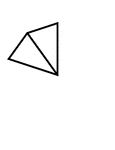### Fractions of 10-sided Polygon

2/10 of a 10 sided polygon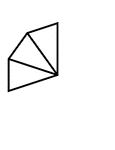### Fractions of 10-sided Polygon

3/10 of a 10 sided polygon